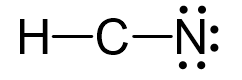## Examples

Carbon is the central atom, so we can draw the skeletal structure:There is a total of 1 + 4 + 5 = 10 valence electrons, and we use four of them to make the bonds.The remaining 6 go on the nitrogen as lone pairs:Because the carbon lacks an octet, we use two lone pairs from the nitrogen to make two additional bonds:

The steric number is 2, and there are no lone pairs on the central atom. Therefore, both the electron and molecular geometries are linear.

Steric number 2 corresponds to sp-hybridization where the bond angles are 180o.